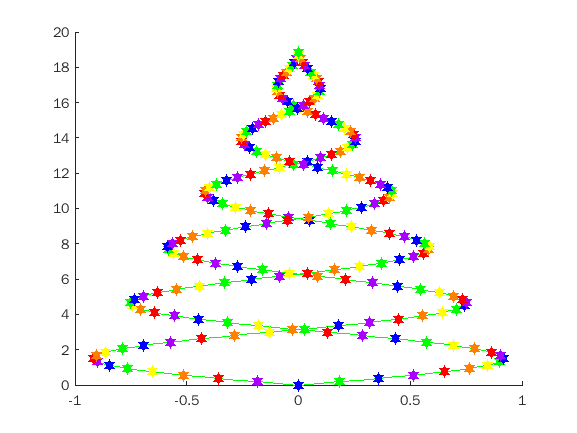• /
• # Better Christmas Tree

on 21 Oct 2021
• 7
• 135
• 0
• 0
• 276
n=203;
x=linspace(0,6*pi,n);
s=linspace(1,0,n);
%Someone informed me the sin looks a lot better than cos
y=sin(x).*s;
r=1:2:n;
hold
Current plot held
plot(y,x,'g',-y,x,'g');
c=repmat((1:6)',17,1);
colormap(prism(6));
t=scatter(y(r),x(r),81,c,'fi','h');
u=scatter(-y(r),x(r),81,c,'fi','h');
% The contest "run" will not peform the animation, but it still fits under
% the 280 character limit. Copy to your own desktop MATLAB installation
% for an amusing little "light show"
for k=1:100
t.CData=circshift(t.CData,1,1);
u.CData=circshift(u.CData,-1,1);
% pause(.05) works better, but is rejected by contest rules
drawnow;
end